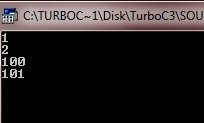# C Enumeration

An enumeration is simply the set of named integer constants. For example, an enumeration of coins used in United States are:

penny, dime, dollar, nickel, quarter

Enumerations are defined like structure. The keyword enum signals the start of an enumeration type.

## Declaring Enumeration in C

Here is the general form to declare enumerations in C language:

`enum tag{enumeration list} variable_list;`

Here, both tag and variable_list are optional (but at least one must be present). Here the following code fragment defines an enumeration called coin :

`enum coin{penny, dime, dollar, quarter, nickel};`

## Declaring Enumeration Variable in C

The enumeration tag name can be used to declare variables of its type. The following C code declares money to be a variable of type coin:

`enum coin money`

Given these declaration, following types of statements are perfectly valid:

```money = dollar
if(money==dime)
{
printf("Money is dime\n");
}```

The key point to understand an enumeration is that each of the symbols stands for an integer value. As such, they can be used anywhere that an integer can be used. And each symbol is given a value one greater than symbol that precedes it. The value of first enumeration symbol is 0. Therefore,

`printf("%d %d", dime, dollar);`

It will displays 0 2 on the screen.

You can specify the value of one or more of the symbols just by using an initializer. Do this by following, the symbol with an equal sign and an integer value. Symbols that appear after an initializer are assigned values greater than preceding value. For example, the following c code assigns the value of 100 to the dime:

`enum coin { nickel, penny, dollar, dime=100, quarter };`

The values of these symbols are :

```nickel		0
penny		1
dollar		2
dime		100
quarter		101```

In C programming, creating code to input and output enumeration symbols is some quite tedious (unless you are willing to settle for their integer values). For example, you need the following code to display, in words, the kind of coin that money contains:

```switch(money)
{
case nickel : printf("nickel");
break;
case penny : printf("penny");
break;
case dollar : printf("dollar");
break;
case dime : printf("dime");
break;
case quarter : printf("quarter");
break;
}```

Sometimes, you also can declare an array of strings and use the enumeration value as an index to translate that value into its corresponding string. For example, the following c code also outputs the proper string:

```char coin_name[] = {
"nickel",
"penny",
"quarter",
"dime",
"dollar"
};```

This only works if no symbol is initialized, because string array must be indexed starting at 0 in strictly ascending order using the increments of 1.

## C Enumeration Example

Here is an example program, demonstrates the enumeration in C language:

```/* C Enumeration - This program demonstrate
* enumeration in C language
*/

#include<stdio.h>
#include<conio.h>
void main()
{
enum coin {penny=1, dime, dollar, quarter=100, nickel};
enum coin money;
clrscr();

money = penny;
printf("%d\n", money);

money = dime;
printf("%d\n", money);

money = quarter;
printf("%d\n", money);

money = nickel;
printf("%d\n", money);

getch();
}```

Here is the sample run of the above C program:Tools
Calculator